Discrete blocks

# Value Accumulator block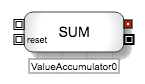The Value Accumulator block accumulates a user-specified numeric value when it is triggered by a discrete event message. After calculating the accumulated value, the block outputs both the accumulated value and the original input discrete event message. You can also attach another discrete event message to the reset input port to reset the block value to 0.

Example Two ValueAccumulator blocks receive fill information. Each accumulates its value based on its internal formula and outputs the calculated value through its numeric output port. Each block can also forward its incoming message to downstream logic.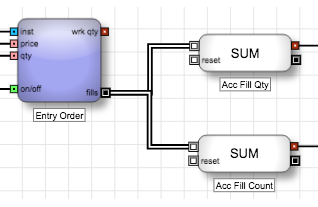You use the Formula Editor for the block to specify the value to accumulate. You can use the value of a field in the message or create a formula that generates a numeric value. For example, if you want accumulate the fill quantity for each fill message attached to the block, you could use the following formula.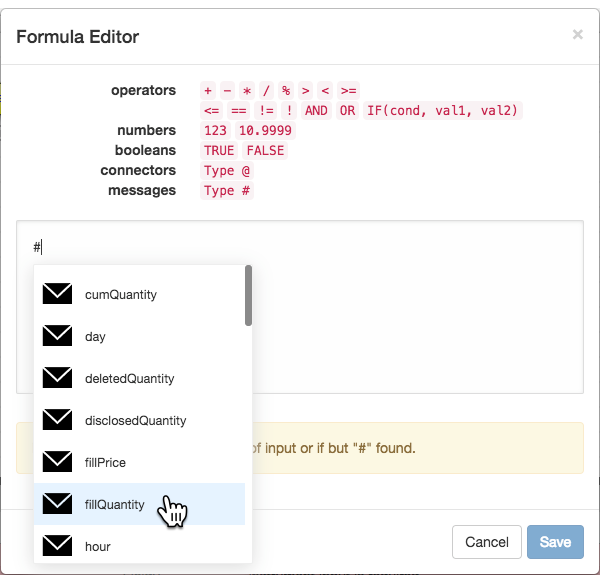In addition to accumulating selected information, such as fill quantity, the Value Accumulator block can also be used as a counter to count the occurrence of a user-defined market event. In the Formula Editor, type the value "1". With this setup, the Value Accumulator will accumulate the numeric value "1" for each occurrence of the event, which results in counting the number of fills.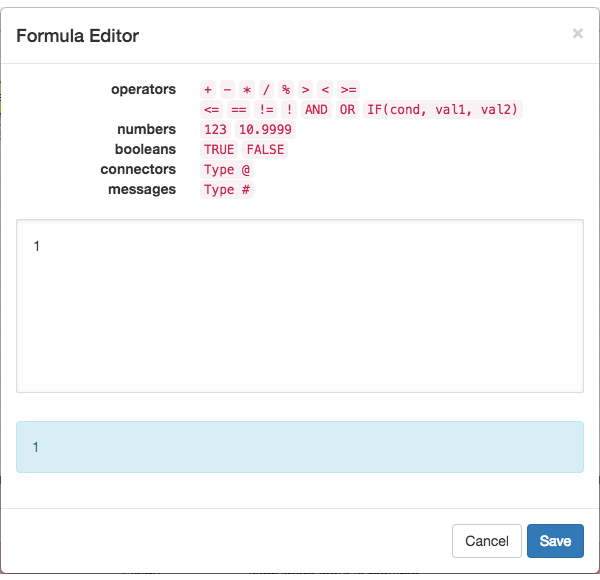Example Disable the Order block when the number of fills reaches three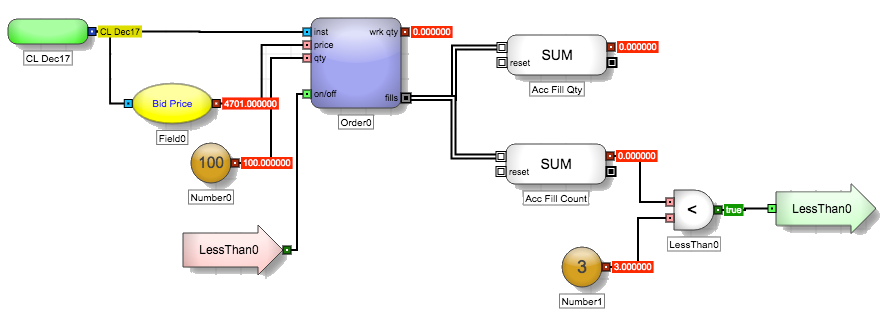## Block properties

To customize the block, update the Block Properties.

Property Description
Name Name to display beneath the block on the ADL canvas
Formula

Equation used to calculate the value of the block

If not yet defined, the field displays an edit link that opens the Formula Editor.